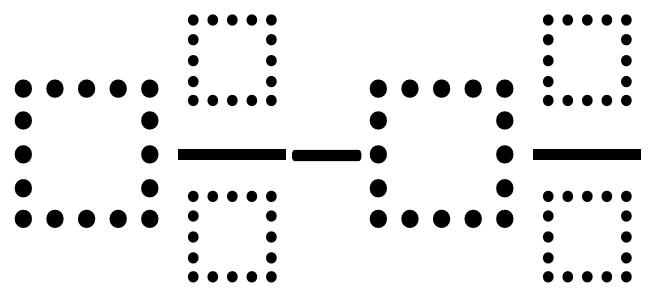# Subtracting Mixed Numbers

Directions: Using the digits 1 to 9 at most one time each, place a digit in each box to make the least possible difference.### Hint

What does the least possible difference mean? (We’re considering this to mean that the two mixed numbers are as close together as possible on a number line)
How do you know you can’t get a lesser difference?

If improper fractions are allowed, many solutions with zero are possible including 7 2/8 – 6 5/4

Source: Robert Kaplinsky

## Add Fractions with Decimal Sums

Directions: Using the digits 1 to 9 at most one time each, place a digit …

1.The smallest difference is not zero. Any negative answer will be smaller than a difference of zero.

The smallest difference I could find is:

( 2 3/7 ) – ( 8 9/1 ) = – 14 5/7

•Hi Robert. I tried to address your concern by revising the hint. What language do you suggest to get it closer to what I want without saying “absolute value” or using “distance” which may be too big of an initial hint?

•Mark Bouwmeester

you might consider taking out the operation all together, and phrase the question as create two numbers that are as ‘almost’ equal. Then extend with explain how you know they are almost equal. This should bring up subtraction as a method for comparison, but could provide some other really interesting discussions as well.

•Robert Kaplinsky

That is a really neat idea Mark. I’ve never tried that but I can see how that could lead to interesting discussions.

•2.( 9 4/6) – (8 5/3)=0

I found it helpful to use the language you suggested, two numbers that are “almost” equal. This prompted me to think about fractions that might be at the same point on a number line (equal), represented differently, thus having a difference of 0.

•Robert Kaplinsky

Yeah, I wonder about being “least helpful” (a la http://robertkaplinsky.com/want-least-helpful-teacher-possible/) with this problem. Initially I don’t talk much about being almost equal. I want them to try and do brute force procedurally to see that this is not an effective way of doing it. Then they are ready to think about it conceptually.

3.I don’t like using improper mixed numbers in the answer. That’s extremely non-standard and poor notation.
If you want that as the answer, you should mention that it’s a “trick question” in the instructions.

The problem is more fair and mathematically interesting if you assume proper mixed numbers, so that the solver needs to discover that they can find two values (with different integer parts) closest in magnitude by making one of them “N + 1/k” and the other “(N-1) + (m-1)/m”, and then figuring which values of k and m are best.

•I totally agree with Mike. No one should be writing a fraction like 6 5/4. I would never accept that from my students.

•Zachary Hocking

I would also agree with Mike and Elizabeth. Having the structure of what a mixed number should look like is part of what will allow students (especially young ones, I think) to engage with the problem.

Similarly, it is this kind of knowledge of structure which would cause students to automatically put the larger value first, thus eliminating the issue Robert Hays brought up, because at this level they are not exposed to negative values.

In a math club type setting, it might start good discussions to “loosen” the rules and see negative answers or improper fractions next to the whole numbers.